# Colleges with the highest SAT scores in Oklahoma

Top 10 colleges in Oklahoma with the highest SAT scores
Looking for the colleges with the highest SAT scores in Oklahoma? Well you're in luck! We've compiled a national college database and have created a list of the top 10 universities with the highest SAT scores in Oklahoma. These are the schools whose applicants had the highest average SAT scores in Oklahoma. And, since these tests are meant to determine academic prowess, they are arguably the schools with the most academically proficient students. You could even say these are the best colleges in Oklahoma. We also include each college's ACT scores and acceptance rate so that you can see where you would have the easiest or hardest time getting in. Read on to find out more.

## University of Tulsa SAT scores

The average SAT score for University of Tulsa is 1300.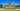The average SAT score of 1300 breaks down into:

• SAT math: 650

The average ACT score for University of Tulsa is 29 and their acceptance rate is 40.8%.

## The University of Oklahoma SAT scores

The average SAT score for The University of Oklahoma is 1210.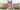The average SAT score of 1210 breaks down into:

• SAT math: 605

The average ACT score for The University of Oklahoma is 26 and their acceptance rate is 80.4%.

## Oklahoma City University SAT scores

The average SAT score for Oklahoma City University is 1200.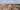The average SAT score of 1200 breaks down into:

• SAT math: 590

The average ACT score for Oklahoma City University is 25 and their acceptance rate is 71.7%.

## Oklahoma State University SAT scores

The average SAT score for Oklahoma State University is 1167.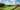The average SAT score of 1167 breaks down into:

• SAT math: 580

The average ACT score for Oklahoma State University is 25 and their acceptance rate is 73.8%.

## Oklahoma Christian University SAT scores

The average SAT score for Oklahoma Christian University is 1160.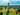The average SAT score of 1160 breaks down into:

• SAT math: 580

The average ACT score for Oklahoma Christian University is 24 and their acceptance rate is 64.8%.

## Southwestern Oklahoma State Univeristy SAT scores

The average SAT score for Southwestern Oklahoma State Univeristy is 1110.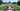The average SAT score of 1110 breaks down into:

• SAT math: 555

The average ACT score for Southwestern Oklahoma State Univeristy is 22 and their acceptance rate is 90.8%.

## Oral Roberts University SAT scores

The average SAT score for Oral Roberts University is 1100.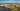The average SAT score of 1100 breaks down into:

• SAT math: 540

The average ACT score for Oral Roberts University is 23 and their acceptance rate is 93.3%.

## Northeastern State University SAT scores

The average SAT score for Northeastern State University is 1080.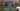The average SAT score of 1080 breaks down into:

• SAT math: 540

The average ACT score for Northeastern State University is 21 and their acceptance rate is 97%.

## Southeastern Oklahoma State University SAT scores

The average SAT score for Southeastern Oklahoma State University is 1080.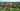The average SAT score of 1080 breaks down into:

• SAT math: 540

The average ACT score for Southeastern Oklahoma State University is 21 and their acceptance rate is 72%.

## University of Central Oklahoma SAT scores

The average SAT score for University of Central Oklahoma is 1080.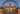The average SAT score of 1080 breaks down into:

• SAT math: 540